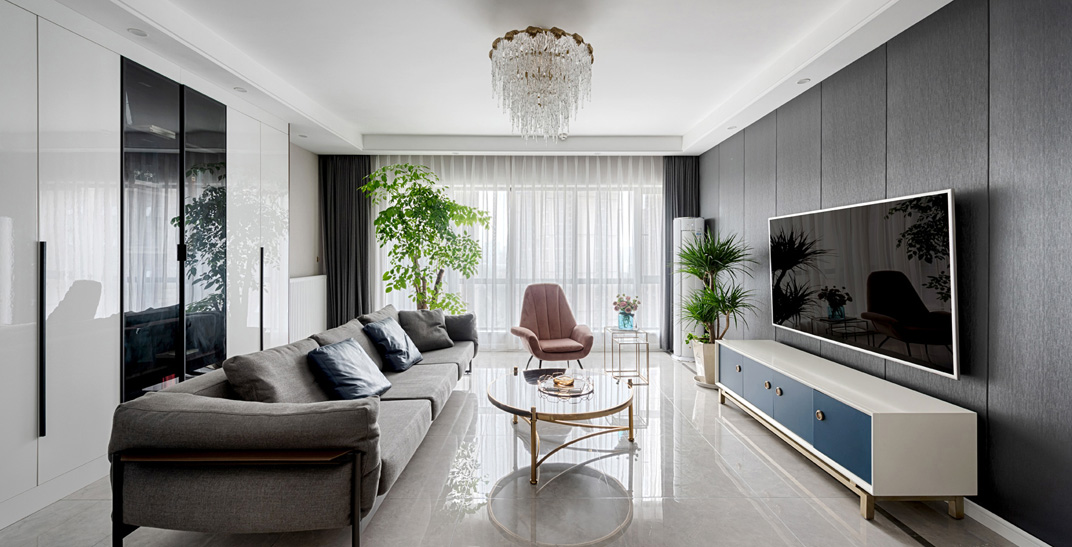----泰戈尔《萤火虫》

客餐厅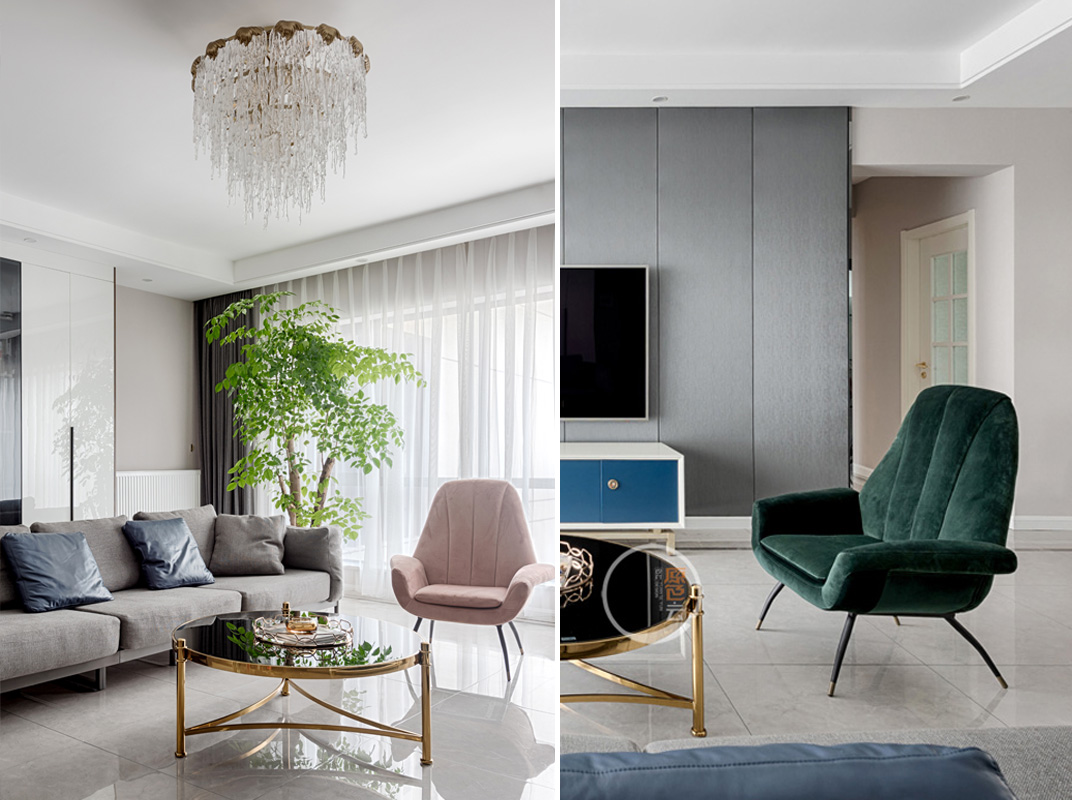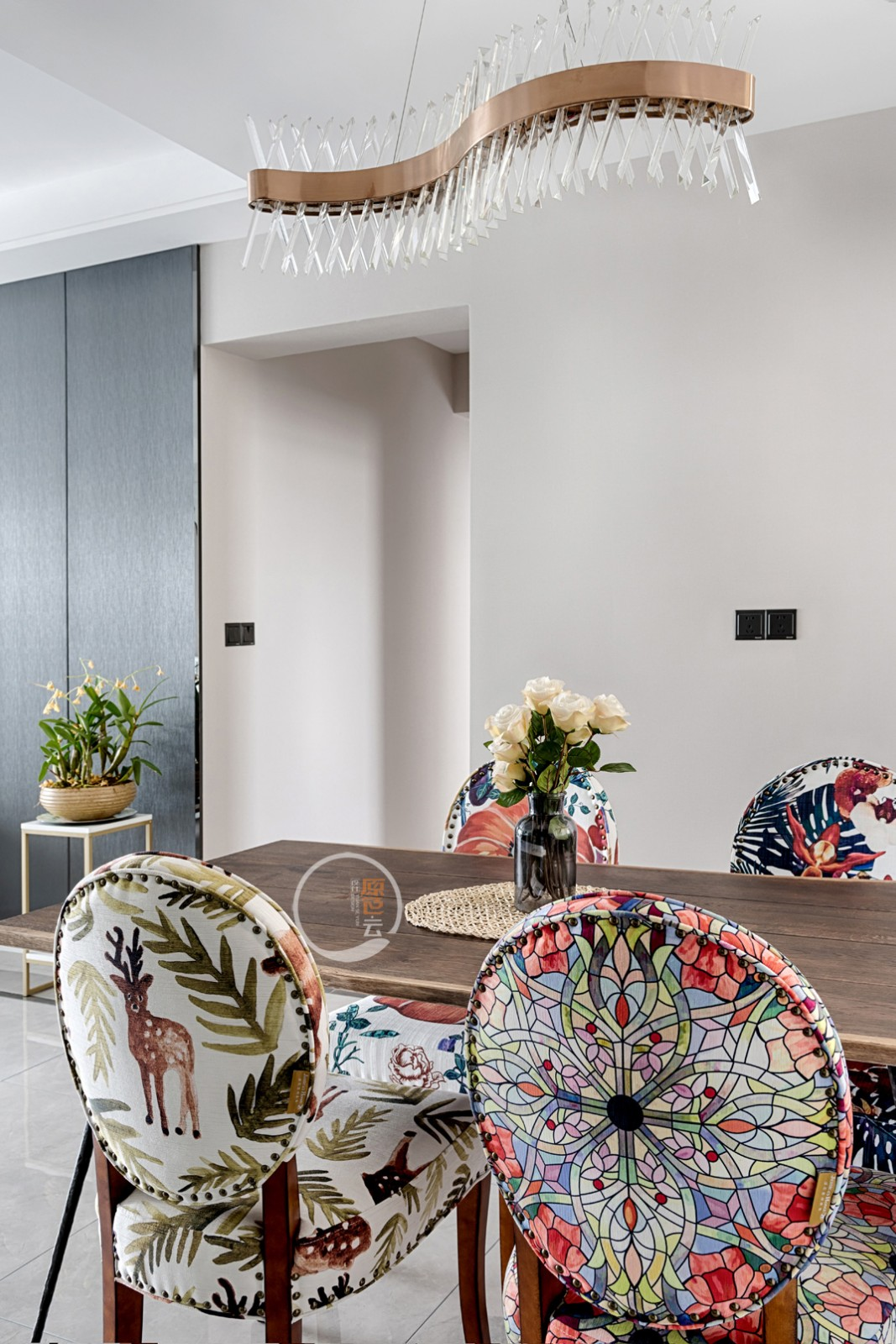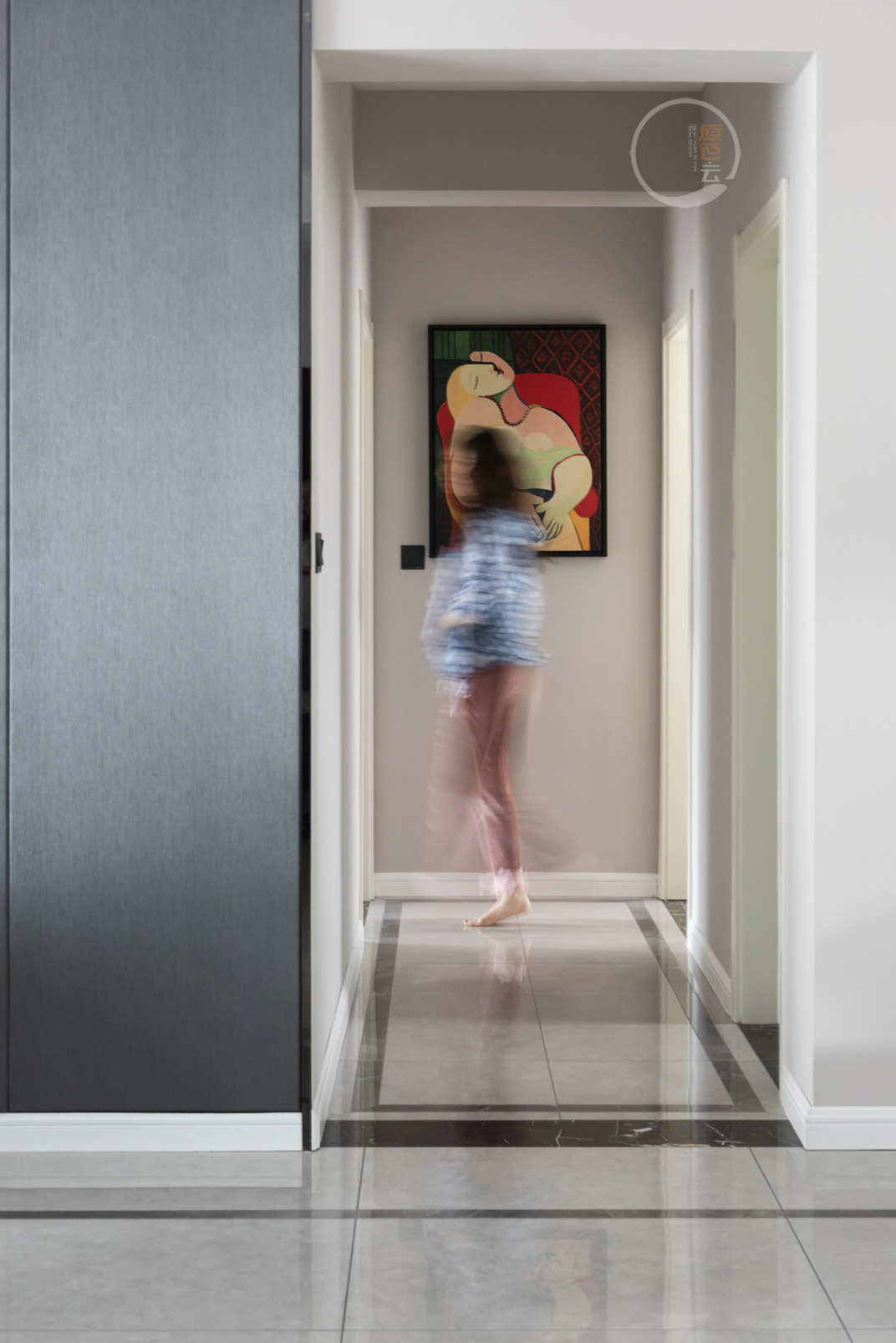女儿房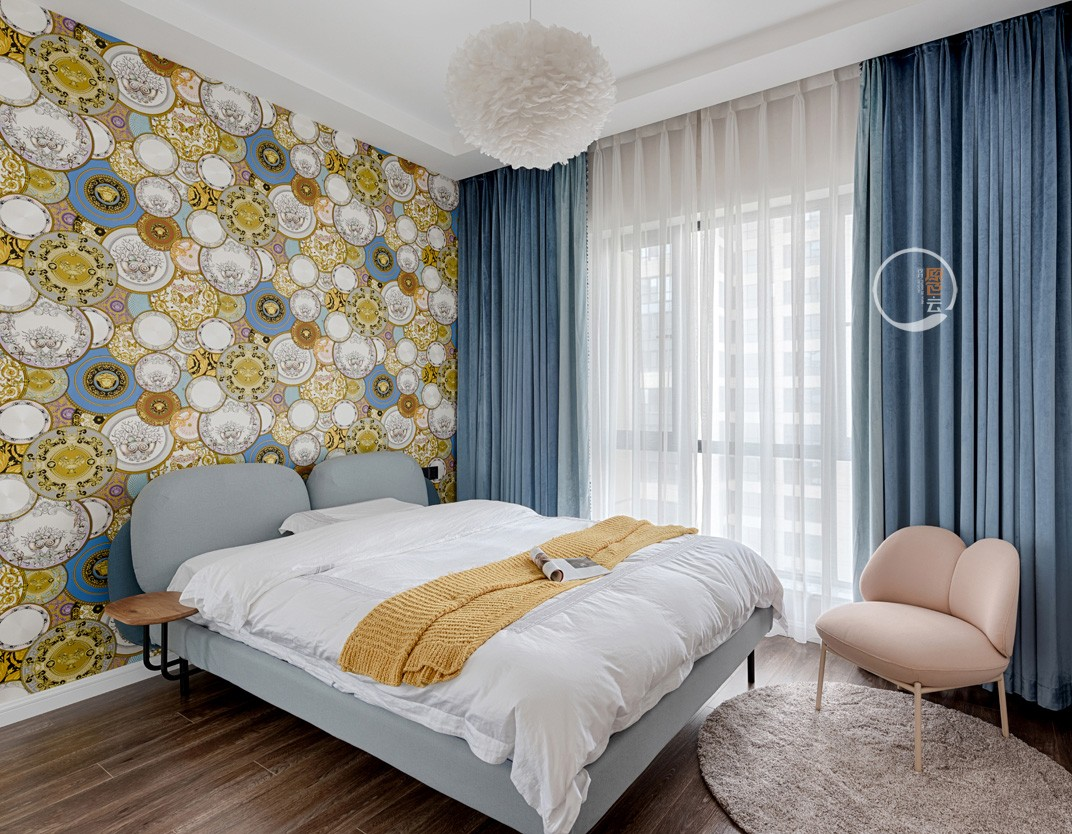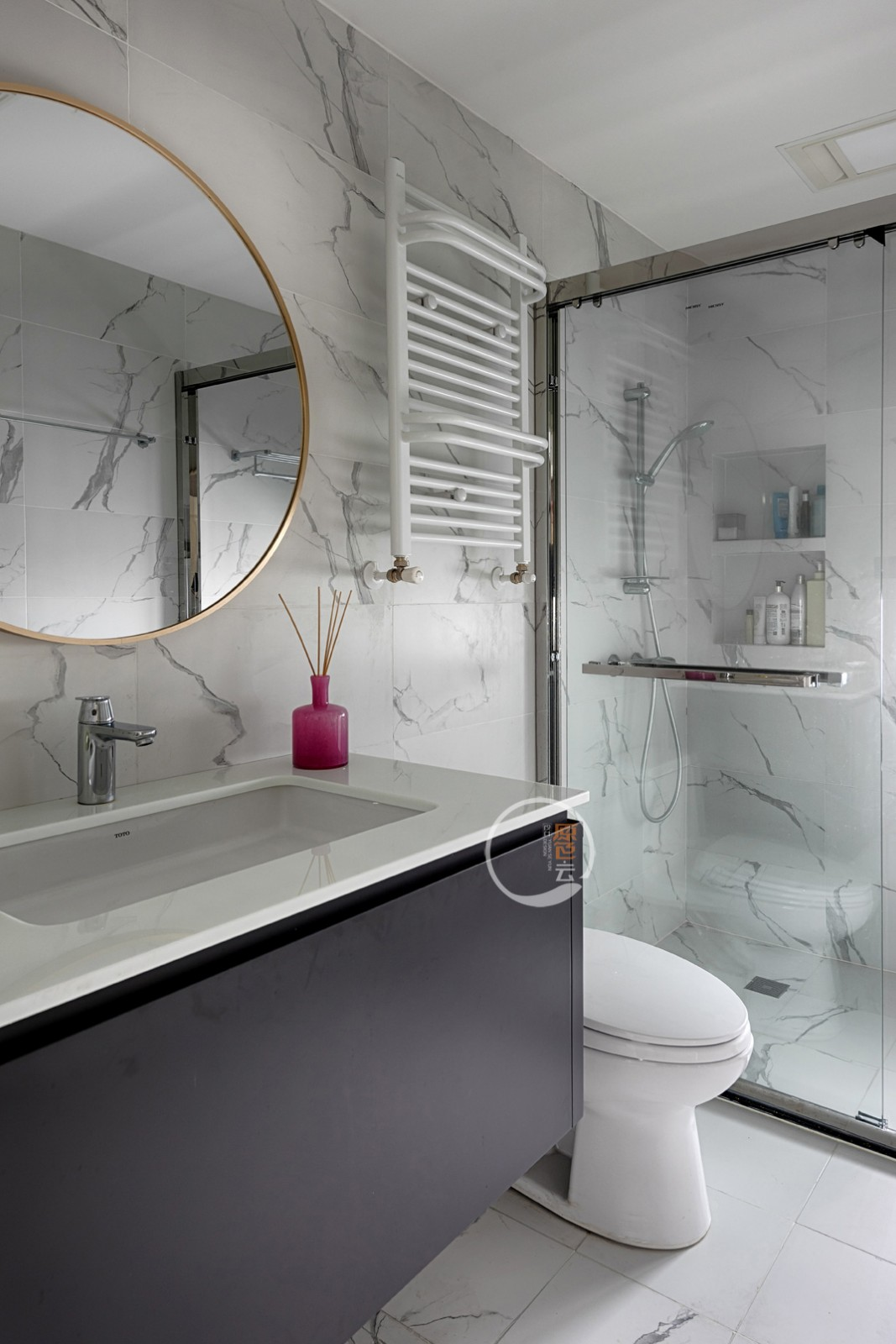<p style="margin-top: 0px; padding: 0px; max-width: 100%; clear: both; min-height: 1em; color: rgb(51, 51, 51); font-family: -apple-system-font, BlinkMacSystemFont, " helvetica="" neue",="" "pingfang="" sc",="" "hiragino="" sans="" gb",="" "microsoft="" yahei="" ui",="" yahei",="" arial,="" sans-serif;="" font-size:="" 17px;="" letter-spacing:="" 0.544px;="" text-align:="" justify;="" white-space:="" normal;="" background-color:="" rgb(255,="" 255,="" 255);="" line-height:="" 25.5px;="" margin-bottom:="" 10px;="" box-sizing:="" border-box="" !important;="" overflow-wrap:="" break-word="" !important;"="">公卫的干湿分区不仅体现在隔断上，颜色上的对比也是很好的区分，整个空间将点、线、面很好的融合。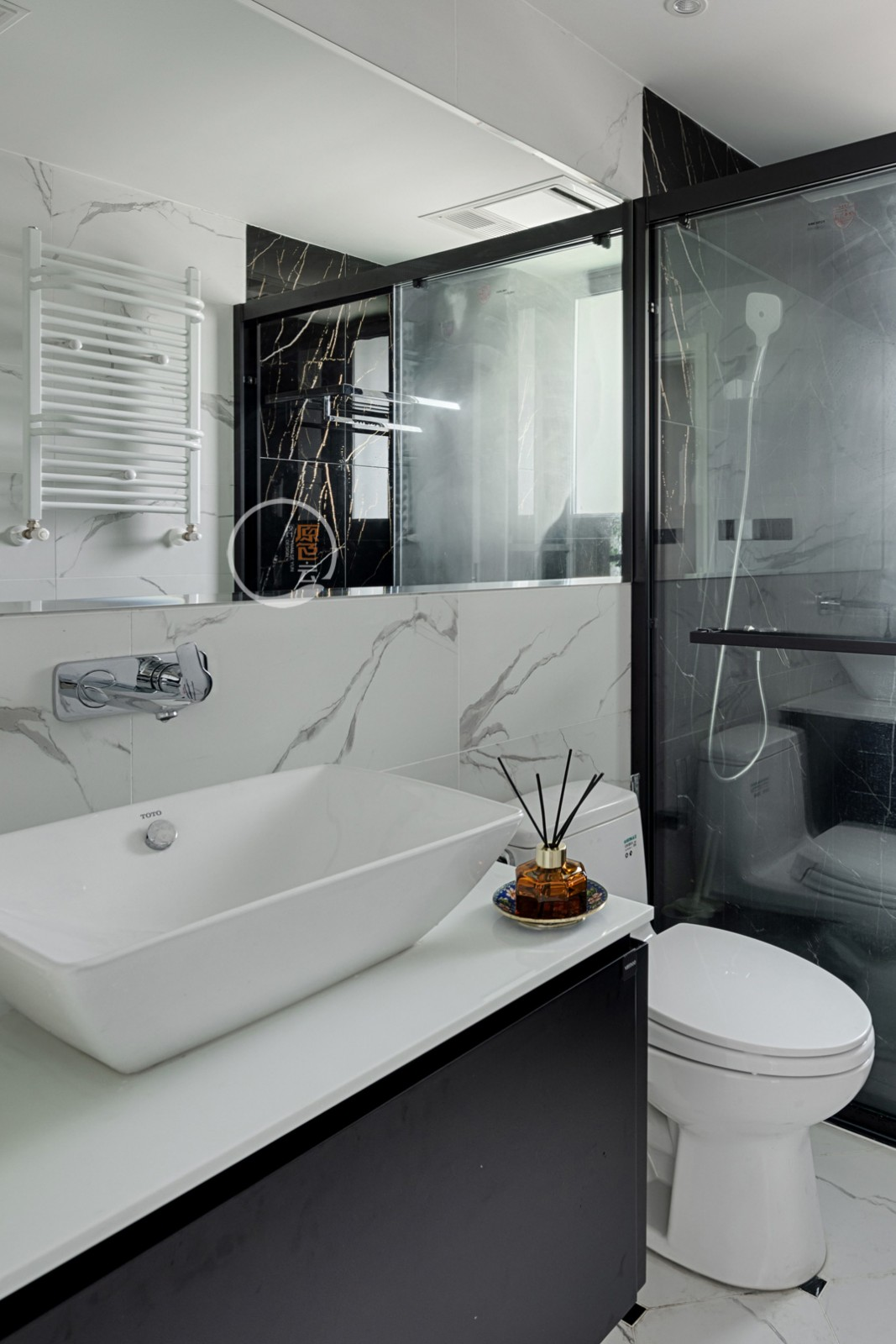厨 房户型图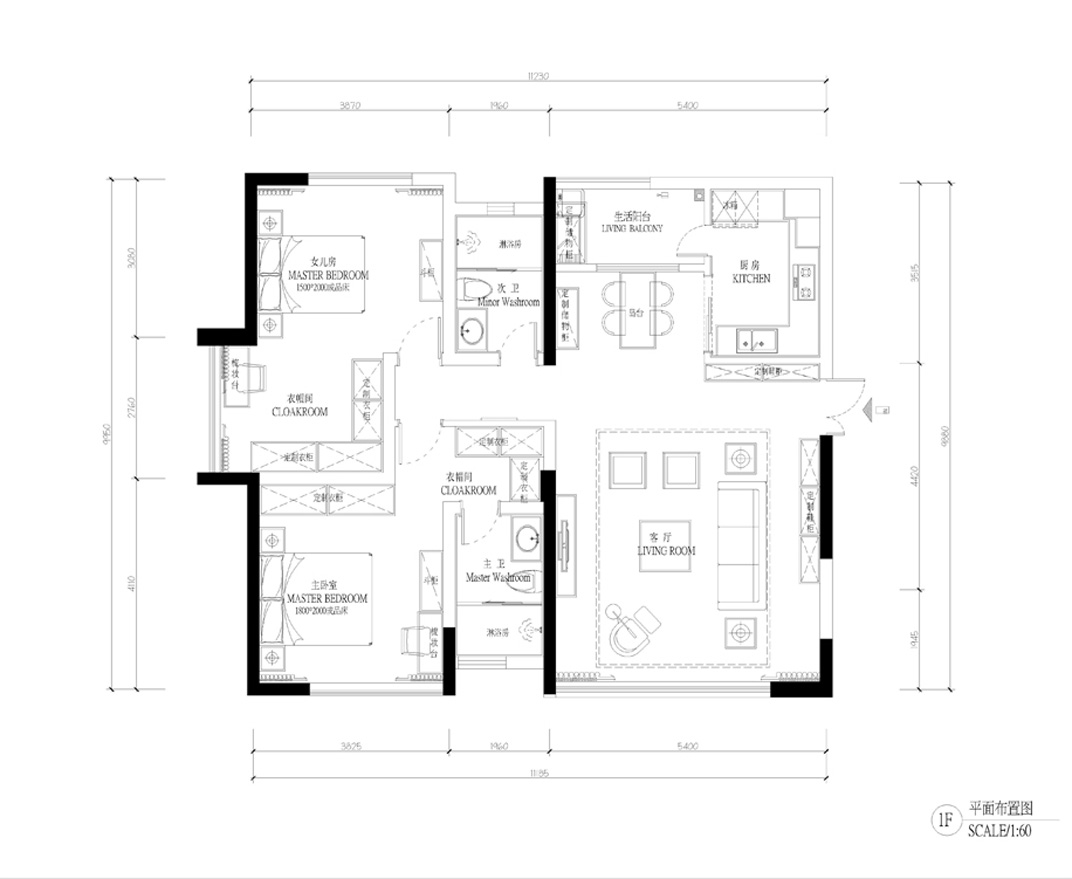24

023-67712306